## Heat Transfer Miscellaneous

#### Heat-Transfer

1. Heat flows through a composite slab, as shown below. The depth of the slab is 1 m. The k values are in W/mK. The overall thermal resistance in K/W is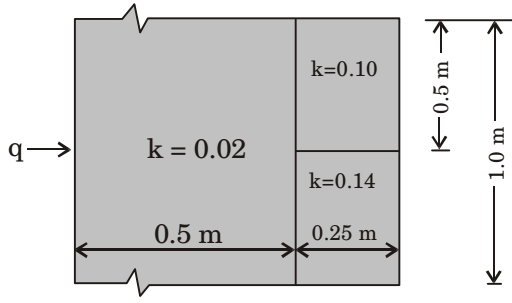1.  L1 = 0.5 = 25 k1 0.02

 2L2 = 5 k2

 2L3 = 12.5 k3

Rtotal = 25 + 3.6 = 28.6 K/W

##### Correct Option: C

 L1 = 0.5 = 25 k1 0.02

 2L2 = 5 k2

 2L3 = 12.5 k3

Rtotal = 25 + 3.6 = 28.6 K/W

1. In a case of one dimensional heat conduction in a medium with constant properties, T is the temperature at position x, at time t.
 Then δT is proportional to δt

1. One dimensional heat conduction equation is,

 δ2T = 1 δT δx2 α δt

 ∴ δ2T ∝ δT δx2 δt

##### Correct Option: D

One dimensional heat conduction equation is,

 δ2T = 1 δT δx2 α δt

 ∴ δ2T ∝ δT δx2 δt

1. A well machined steel plate of thickness L is kept such that the wall temperature are Th and Tc as seen in the figure below. A smooth copper plate of the same thickness L is now attached to the steel plate without any gap as indicated in the figure below. The temperature at the interface is Tt. The temperatures of the outer walls are still the same at Th and Tc. The heat transfer rates are q1 and q2 per unit area in the two cases respectively in the direction shown. Which of the following statements is correct?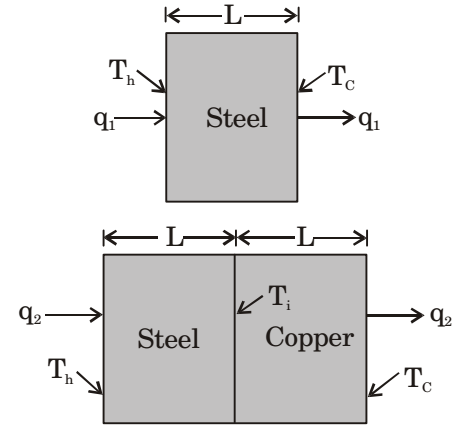1.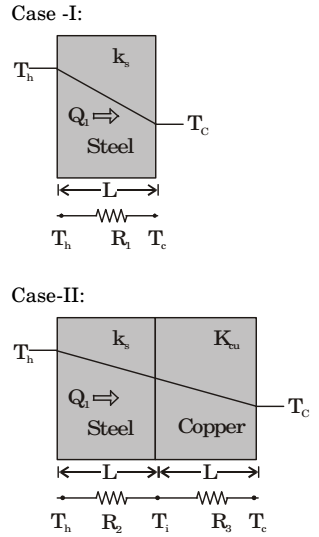For caseI-:

 Q1 = Th - Tc R1

 where R1 = L KSA

For Case-II:
 Q2 = Th - Tc R2 + R3

 where R2 = L and R3 = L KsA KcuA

As R2 + R3 > R1 at constant temperature difference in both cases.
 Then q1or Q1> q2or Q2A A

For Case-II:
R2 > R3
∵ kcu > ks
 Q2 = Th - T1 R2

 or R2 = Th - T1 Q2

 also Q2 = Ti - Tc R3

 or R3 = Ti - Tc Q2

 ∴ Th - Ti > Ti - Tc Q2 Q2

or Th – Ti > Ti – Tc
or Th + Tc > 2Ti
 or Ti < Th + Tc 2

##### Correct Option: DFor caseI-:

 Q1 = Th - Tc R1

 where R1 = L KSA

For Case-II:
 Q2 = Th - Tc R2 + R3

 where R2 = L and R3 = L KsA KcuA

As R2 + R3 > R1 at constant temperature difference in both cases.
 Then q1or Q1> q2or Q2A A

For Case-II:
R2 > R3
∵ kcu > ks
 Q2 = Th - T1 R2

 or R2 = Th - T1 Q2

 also Q2 = Ti - Tc R3

 or R3 = Ti - Tc Q2

 ∴ Th - Ti > Ti - Tc Q2 Q2

or Th – Ti > Ti – Tc
or Th + Tc > 2Ti
 or Ti < Th + Tc 2

1. A stainless steel tube (ks = 19 W/mK) of 2 cm ID and 5 cm OD is insulated with 3 cm thick asbestos (ka = 0.2 W/mK). If the temperature difference between the inner most and outermost surfaces is 600°C, the heat transfer rate per unit length is

1. Given: d1 = 2 cm
r1 = 1 cm
d2 = 5 cm
r2 = 2.5 cm
d3 = 5 + 2 × 3 = 11
r3 = 5.5 cm
Equivalent thermal resistance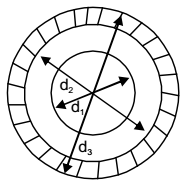Rt = 1 In r2 + 1 In r3 2πkst r1 2πka r2

 = 1 In 2.5 + 1 In 5.5 2π × 19 1 2π × 0.2 2.5

= 7.67 × 10–3 + 0.627 = 0.635
∴ Heat transfer rate per unit length
 t1 - t2 = 600 = 944.88 W/m Rt 0.635

##### Correct Option: C

Given: d1 = 2 cm
r1 = 1 cm
d2 = 5 cm
r2 = 2.5 cm
d3 = 5 + 2 × 3 = 11
r3 = 5.5 cm
Equivalent thermal resistanceRt = 1 In r2 + 1 In r3 2πkst r1 2πka r2

 = 1 In 2.5 + 1 In 5.5 2π × 19 1 2π × 0.2 2.5

= 7.67 × 10–3 + 0.627 = 0.635
∴ Heat transfer rate per unit length
 t1 - t2 = 600 = 944.88 W/m Rt 0.635

1. One dimensional unsteady state heat transfer equation for a sphere with heat generation at the rate of q can be written

1.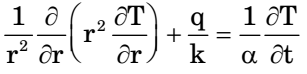##### Correct Option: B